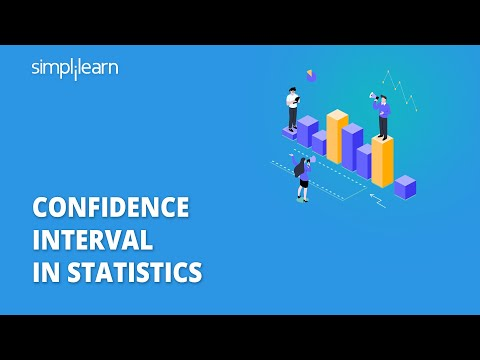A confidence interval is a type of interval calculation in statistics derived from observed data and holds the actual value of an unknown parameter. It's linked to the confidence level, which measures how confident the interval is in estimating the deterministic parameter.

#### Post Graduate Program in Data Analytics

In partnership with Purdue University## What Is Confidence Interval?

A confidence interval shows the probability that a parameter will fall between a pair of values around the mean. Confidence intervals show the degree of uncertainty or certainty in a sampling method. They are constructed using confidence levels of 95% or 99%.## What Does a 95% Confidence Interval Mean?

The 95% confidence interval is the range that you can be 95% confident that the similarly constructed intervals will contain the parameter being estimated. The sample mean (center of the CI) will vary from sample to sample because of natural sampling variability.

Statisticians use confidence intervals to measure the uncertainty in a sample variable. The confidence is in the method, not in a particular CI. Approximately 95% of the intervals constructed would capture the true population mean if the sampling method was repeated many times.

## Confidence Interval Formula

The formula to find Confidence Interval is:• X bar is the sample mean.
• Z is the number of standard deviations from the sample mean.
• S is the standard deviation in the sample.
• n is the size of the sample.

The value after the ± symbol is known as the margin of error.

Question: In a tree, there are hundreds of mangoes. You randomly choose 40 mangoes with a mean of 80 and a standard deviation of 4.3. Determine that the mangoes are big enough.

Solution:

Mean = 80

Standard deviation = 4.3

Number of observations = 40

Take the confidence level as 95%. Therefore the value of Z = 1.9

Substituting the value in the formula, we get

= 80 ± 1.960 × [ 4.3 / √40 ]

= 80 ± 1.960 × [ 4.3 / 6.32]

= 80 ± 1.960 × 0.6803

= 80 ± 1.33

The margin of error is 1.33

All the hundreds of mangoes are likely to be in the range of 78.67 and 81.33.

#### FREE Course: Introduction to Data Analytics

Learn Data Analytics Concepts, Tools & Skills## The Z-Value

Z is the number of standard deviations from the sample mean (1.96 for 95% confidence, 2.576 for 99%). Z-scores can be positive or negative. The sign tells you whether the observation is above or below the mean. For example, a z-score of +1 shows that the data point falls one standard deviation above the mean, while a -1 signifies it is one standard deviation below the mean. A z-score of zero equals the mean.

## How Are Confidence Intervals Used?

Statisticians use confidence intervals to measure the uncertainty in a sample variable. For instance, a researcher may randomly select different samples from the same population and compute a confidence interval for each sample to determine how well it represents the actual value of the population variable. The resulting datasets are all different, with some intervals included and others not including the true population parameter.## What Is a T-Test?

Statistical methods such as the T-Test are used to calculate confidence intervals. A t-test is an inferential statistic used to observe a significant difference in the average of two groups that could be linked to specific characteristics. Three fundamental data values are required to calculate a t-test. They include the mean difference (the difference between the mean values in each data set), the standard deviation of each group, and the data points in each group.

Looking forward to a career in Data Analytics? Check out the Data Analytics Cetification Training and get certified today.

## Conclusion

In this confidence interval in statistics tutorial, you have learned the importance of confidence intervals and the formula to calculate the same. The confidence interval tells you the range of values you can expect if you re-do the experiment in the same way.

If you are looking to pursue this further and make a career as a Data Analyst, Simplilearn’s Data Analytics PGP in partnership with Purdue University & in collaboration with IBM is the program for you.Kartik Menon